# pie3D function in R

Jim Lemon

## Basic 3D pie chart

3D pie charts are not recommended, but if you really want to create them you can use `pie3D` from `plotrix` package. The default 3D pie chart will look like the following.

``````# install.packages("plotrix")
library(plotrix)

data <- c(19, 21, 54, 12, 36, 12)

pie3D(data)``````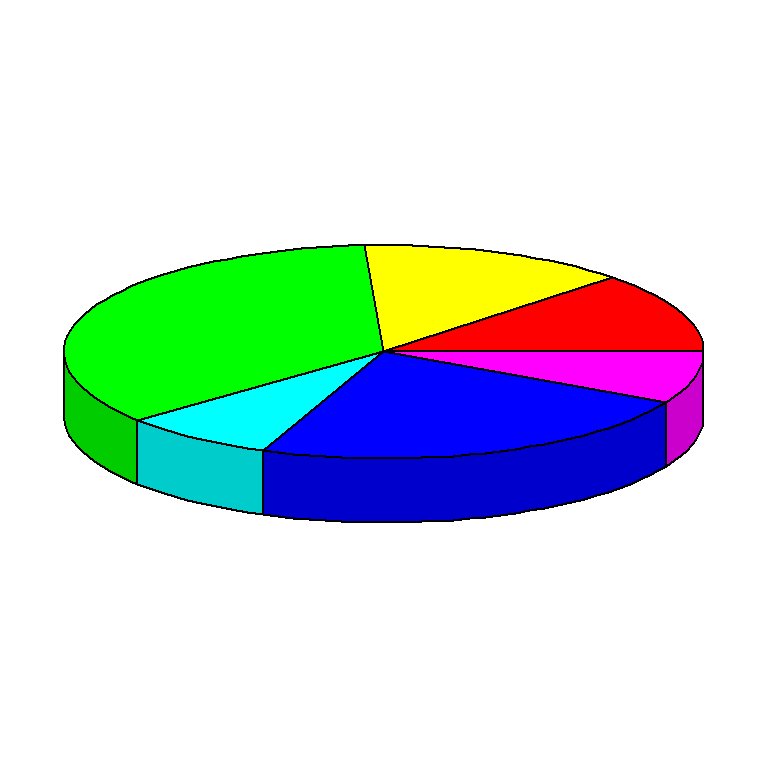The `radius` argument allows modifying the radius of the pie in user units. Default value is 1.

``````# install.packages("plotrix")
library(plotrix)

data <- c(19, 21, 54, 12, 36, 12)

pie3D(data,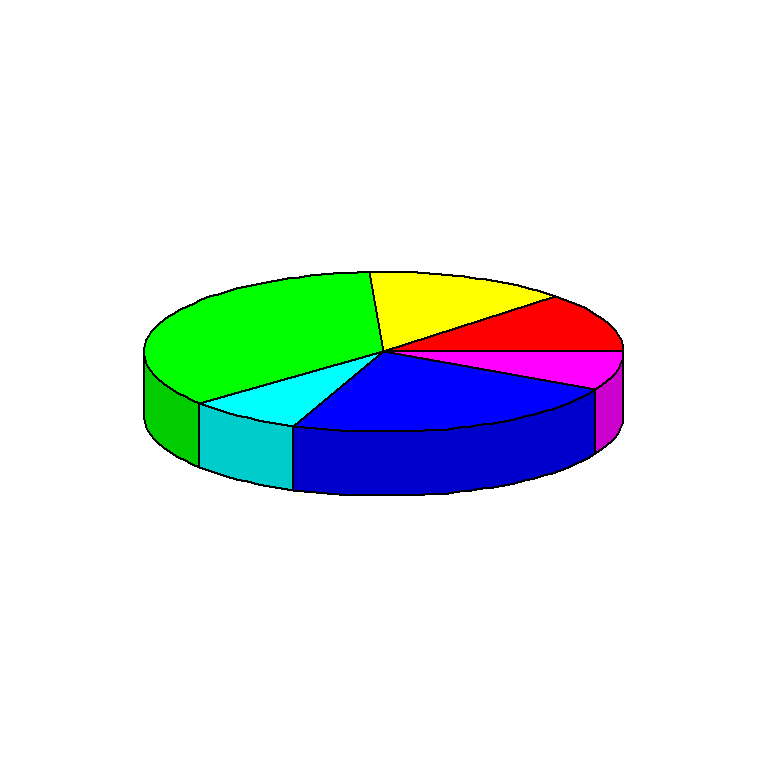Height

Similarly, you can change the height of the pie with `height`. Default value is 0.1

``````# install.packages("plotrix")
library(plotrix)

data <- c(19, 21, 54, 12, 36, 12)

pie3D(data,
height = 0.2)``````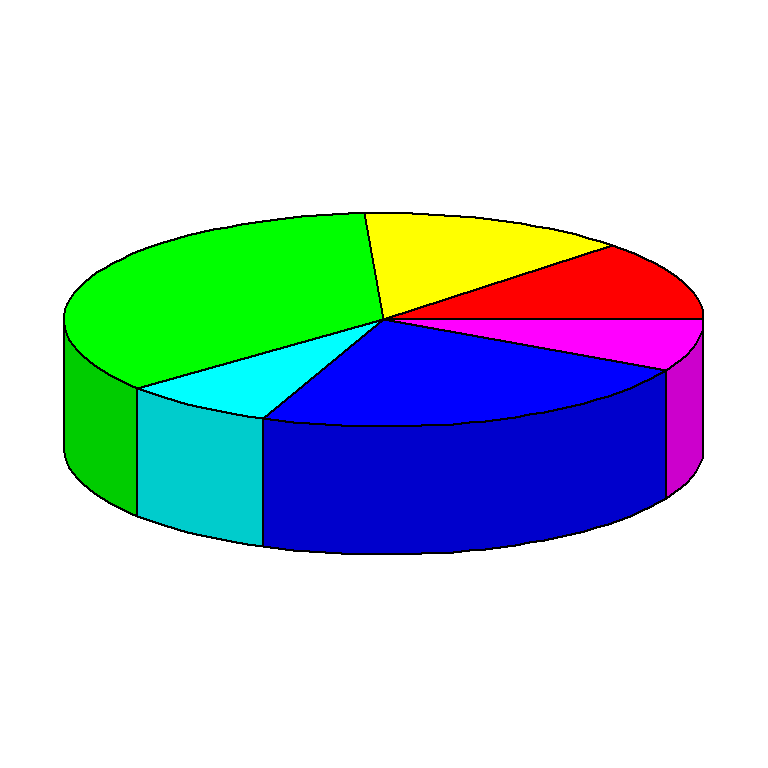Angle

You can also change the viewing angle with `theta`. Default value is pi/6.

``````# install.packages("plotrix")
library(plotrix)

data <- c(19, 21, 54, 12, 36, 12)

pie3D(data,
theta = 1.5)``````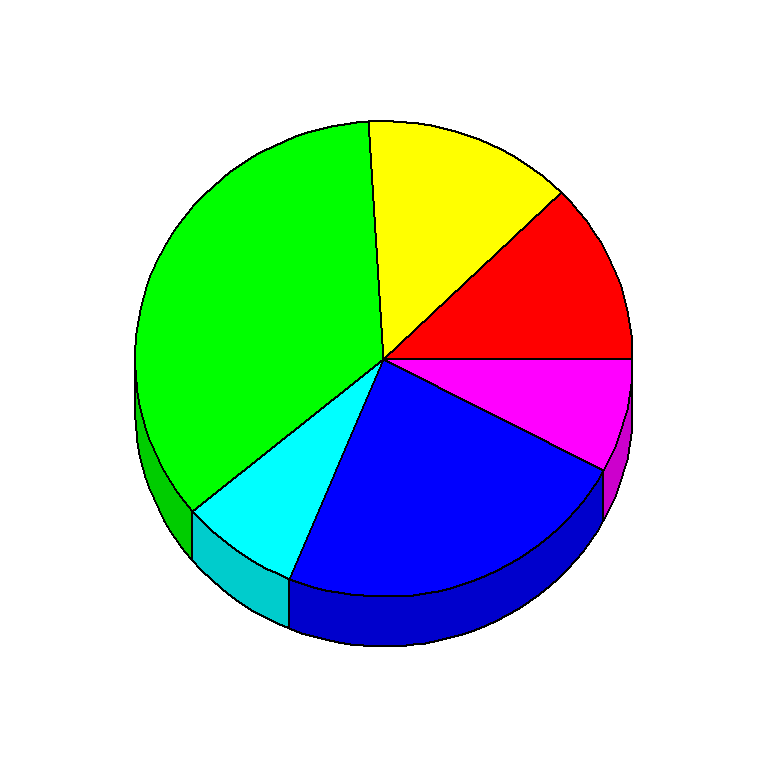## `pie3D` color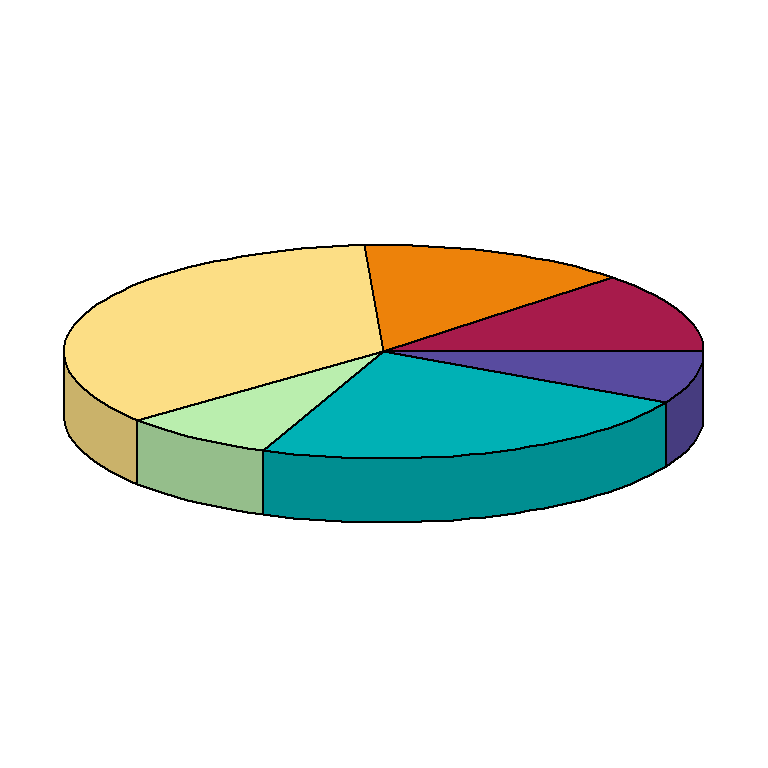The color of the pie slices can be customized with `col`. In the following examples we are using the `"Spectral"` palette, generating as many colors as slices.

``````# install.packages("plotrix")
library(plotrix)

data <- c(19, 21, 54, 12, 36, 12)

pie3D(data,
col = hcl.colors(length(data), "Spectral"))``````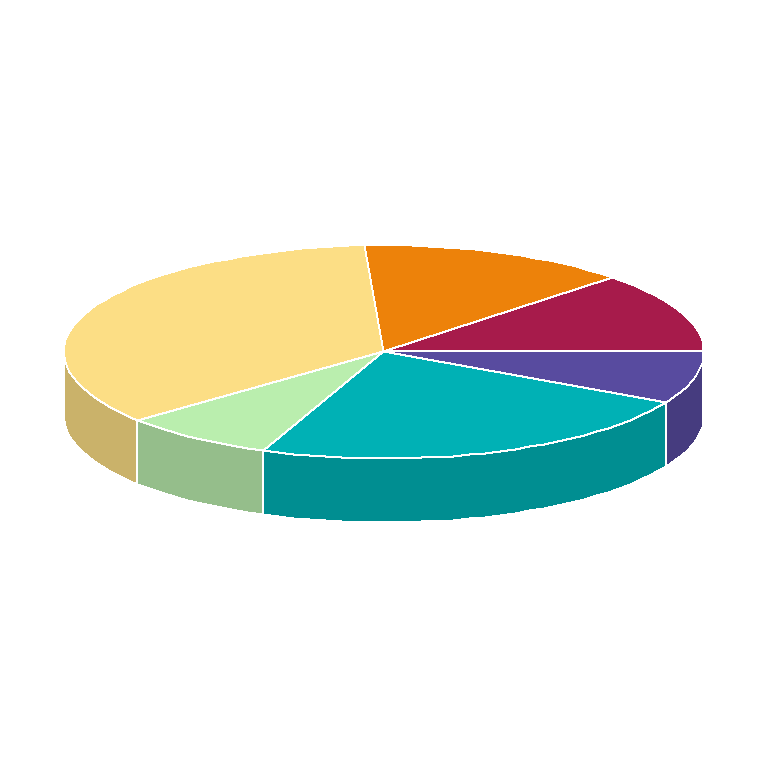The border color can also be customized with `border` argument. In the following block of code we are setting a white border.

``````# install.packages("plotrix")
library(plotrix)

data <- c(19, 21, 54, 12, 36, 12)

pie3D(data,
col = hcl.colors(length(data), "Spectral"),
border = "white")``````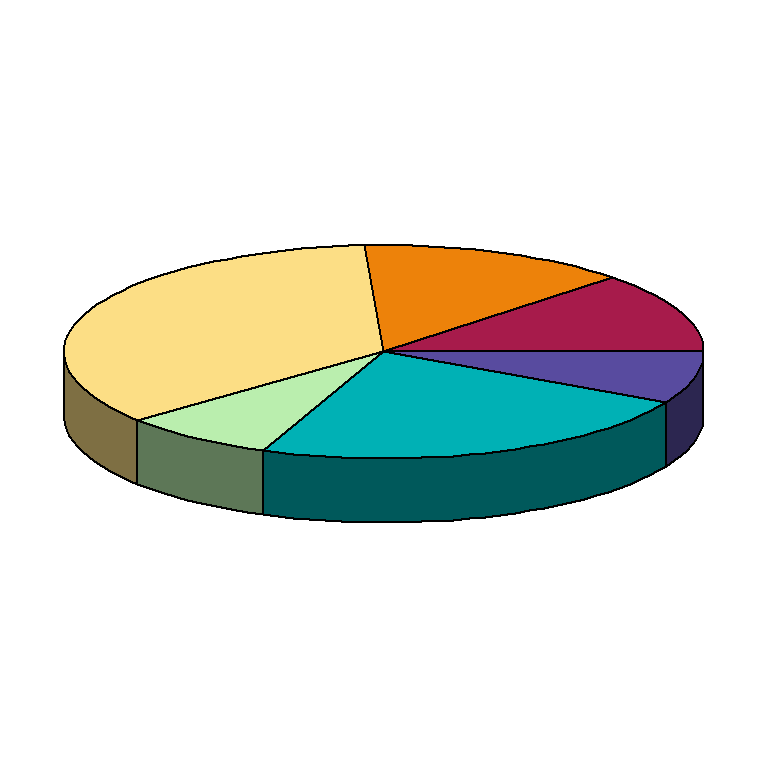Finally, you can change the shade of the border of the pie, in order to modify the 3D effect. This will darker or lighter the corresponding colors.

``````# install.packages("plotrix")
library(plotrix)

data <- c(19, 21, 54, 12, 36, 12)

pie3D(data,
col = hcl.colors(length(data), "Spectral"),

## `pie3D` labels

You can add labels to the pie representing the value, label or percentage for each slice passing a vector of the same size as the number of slices to `labels` argument.

``````# install.packages("plotrix")
library(plotrix)

data <- c(19, 21, 54, 12, 36, 12)

pie3D(data,
col = hcl.colors(length(data), "Spectral"),
labels = data)``````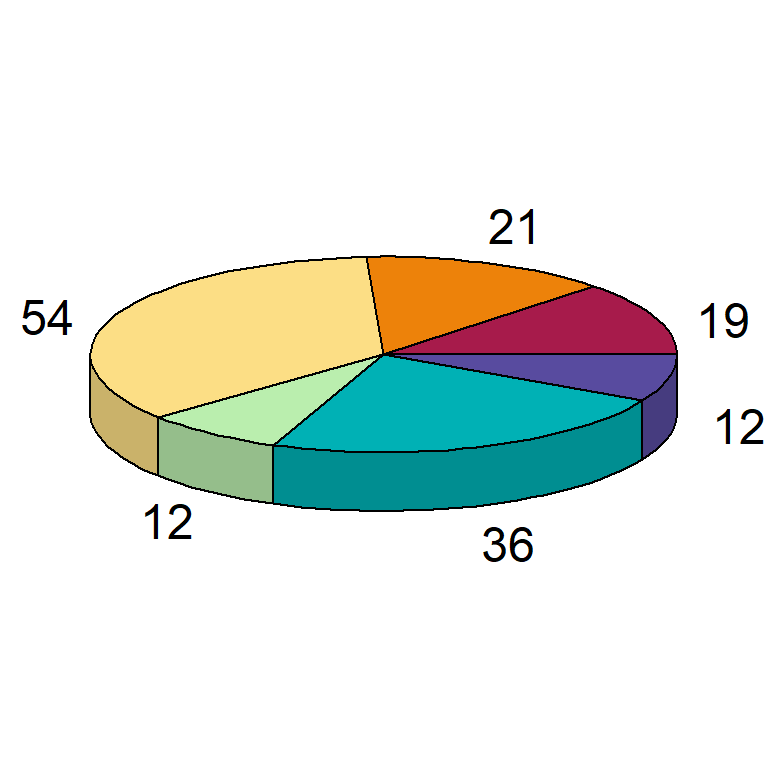If you have a numeric vector that doesn’t represent percentages consider transforming the data into percentage as in this example.

``````# install.packages("plotrix")
library(plotrix)

data <- c(19, 21, 54, 12, 36, 12)
lab <- paste0(round(data/sum(data) * 100, 2), "%")

pie3D(data,
col = hcl.colors(length(data), "Spectral"),
labels = lab)``````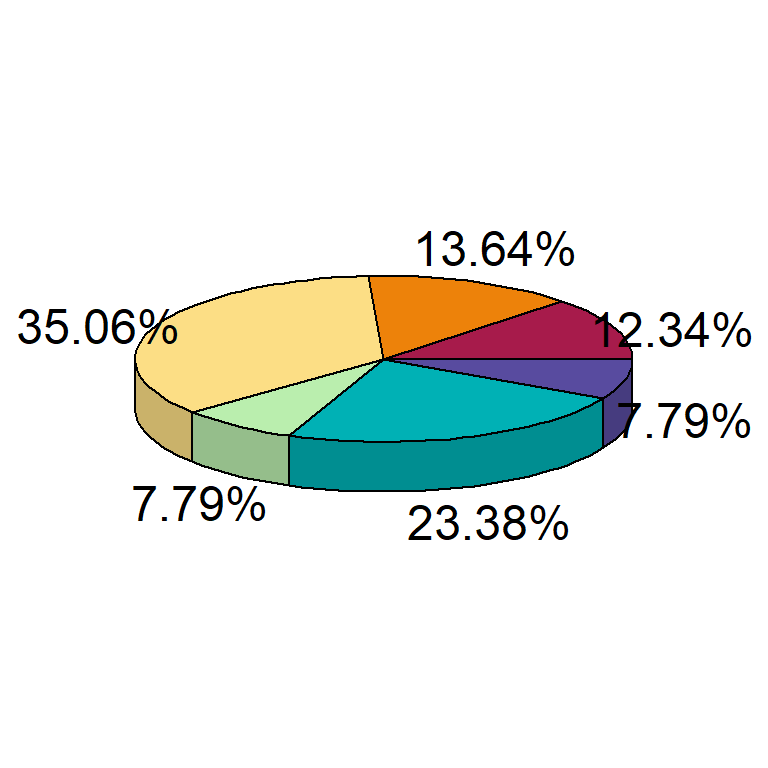The color and size of the labels can be customized with `labelcol` and `labelcex` arguments. The latter defaults to 1.5.

``````# install.packages("plotrix")
library(plotrix)

data <- c(19, 21, 54, 12, 36, 12)

pie3D(data,
col = hcl.colors(length(data), "Spectral"),
labels = data,
labelcol = "red",
labelcex = 0.75)``````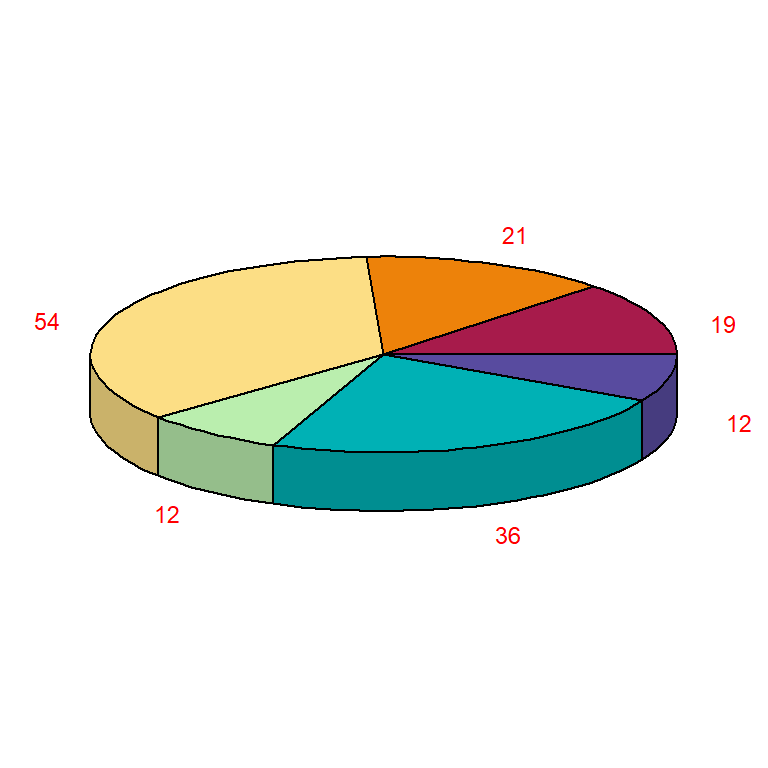## Explode pie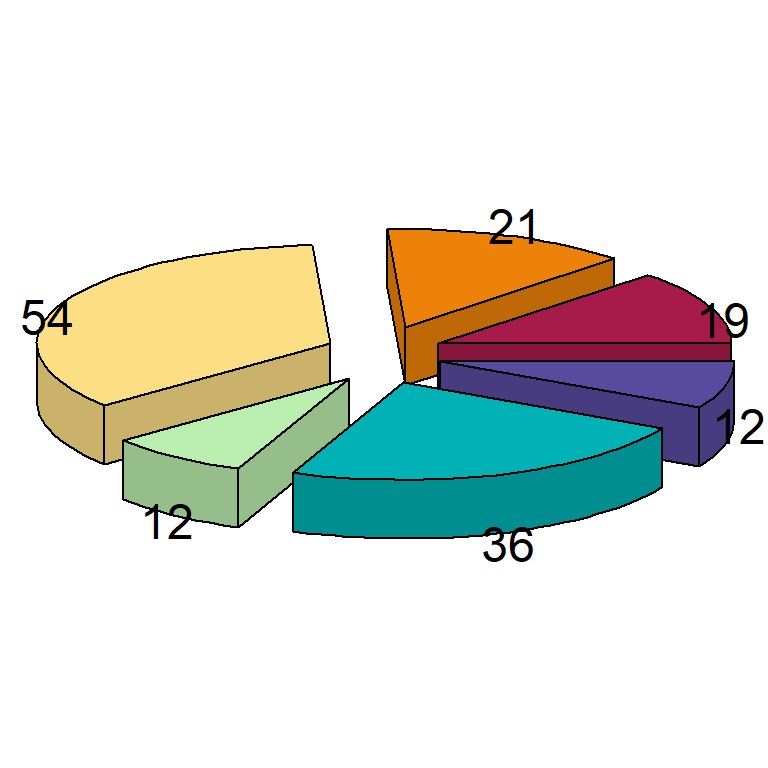You can also “explode” the pie with `explode`.

``````# install.packages("plotrix")
library(plotrix)

data <- c(19, 21, 54, 12, 36, 12)

pie3D(data, mar = rep(1.75, 4),
col = hcl.colors(length(data), "Spectral"),
labels = data,
explode = 0.2)``````